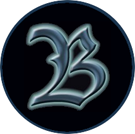Jump to content## Recommended Posts

{{ Personaggio
| Nome = Walder
| Cognome = Rivers
| Soprannomi = Walder il Bastardo
}}

[[Walder Rivers]], detto "Walder il Bastardo", è un figlio illegittimo di [[Walder Frey (il Ritardatario)|Lord Walder Frey]] delle [[Torri Gemelle]].<ref name="ACOKAH">[[ACOK - Appendici#Appendice H|ACOK - Appendice H]]</ref>

__TOC__

== Famiglia e Genealogia ==
<table style="border-collapse:collapse;">
{{Genealogia | Quantità = 9 | Arg1 = C | Arg2 = A | Arg3 = H | Arg4 = A | Arg5 = C | Arg6 = A | Arg7 = H | Arg8 = A | Arg9 = C | Nome2 = ??? | Nome4 = ??? | Nome6 = ??? | Nome8 = ???}}
{{Genealogia | Quantità = 8 | Arg1 = E | Arg2 = C | Arg3 = I | Arg4 = F | Arg5 = C | Arg6 = I | Arg7 = C | Arg8 = E}}
{{Genealogia | Quantità = 15 | Arg1 = D | Arg2 = C | Arg3 = B | Arg4 = A | Arg5 = T | Arg6 = S | Arg7 = S | Arg8 = J | Arg9 = S | Arg10 = S | Arg11 = T | Arg12 = A | Arg13 = B | Arg14 = C | Arg15 = D | Nome4 = [[Walder Frey (il Ritardatario)|Walder Frey]] | Nome12 = ???}}
{{Genealogia | Quantità = 5 | Arg1 = F | Arg2 = D | Arg3 = I | Arg4 = F | Arg5 = D}}
{{Genealogia | Quantità = 8 | Arg1 = F | Arg2 = B | Arg3 = A | Arg4 = H | Arg5 = A | Arg6 = D | Arg7 = C | Arg8 = B | Nome3 = [[Walder Rivers]] | Nome5 = ???}}
{{Genealogia | Quantità = 11 | Arg1 = F | Arg2 = C | Arg3 = B | Arg4 = K | Arg5 = 2 | Arg6 = N | Arg7 = L | Arg8 = M | Arg9 = B | Arg10 = E | Arg11 = C}}
{{Genealogia | Quantità = 8 | Arg1 = F | Arg2 = B | Arg3 = A | Arg4 = C | Arg5 = A | Arg6 = D | Arg7 = C | Arg8 = B | Nome3 = [[Aemon Rivers]] | Nome5 = [[Walda Rivers]]}}
</table>

== Note ==
<references />

{{Portale | Argomento1 = Personaggi}}

<noinclude>[[Categoria:Personaggi]] [[Categoria:Regno di Fiumi]] [[Categoria:Terre dei Fiumi]] [[Categoria:WP]]</noinclude>

• Replies 1
• Created
• Last Reply

#### Popular Days

Uppato e fatto il redirect.

Ho aggiunto anche lo stemma personale visto che è pronto.## Create an account or sign in to comment

You need to be a member in order to leave a comment

## Create an account

Sign up for a new account in our community. It's easy!

Register a new account

## Sign in

Already have an account? Sign in here.

Sign In Now

• ### Upcoming Events

• 14 April 2021
Until
14 May 2021

• 23 April 2021 07:00 PM
• 27 April 2021 06:59 PM
• ### Member Statistics

• Total Members
5,838
• Most Online
1,394

• ### Forum Statistics

• Total Topics
15,977
• Total Posts
734,930
×

• #### Activity

×
• Create New...